# GRE Quantitative Reasoning - Coordinate Geometry: Tutoring Solution Chapter Exam

Exam Instructions:

Choose your answers to the questions and click 'Next' to see the next set of questions. You can skip questions if you would like and come back to them later with the yellow "Go To First Skipped Question" button. When you have completed the practice exam, a green submit button will appear. Click it to see your results. Good luck!

### Page 1

#### Question 1 1. What are the coordinates of this point?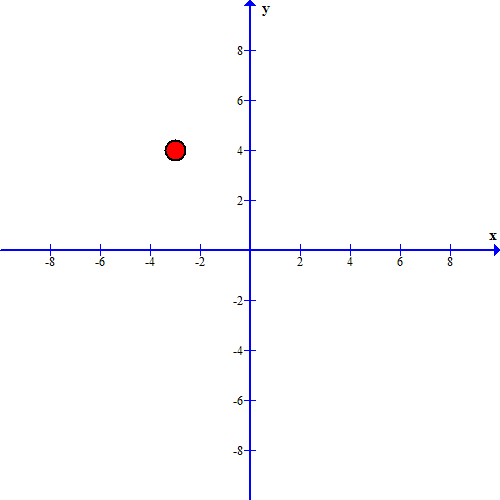#### Question 3 3. Estimate the coordinates of the midpoint of the line segment below: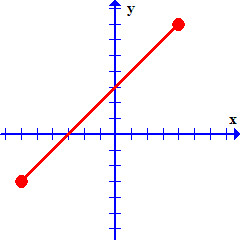### Page 2

#### Question 9 9. Which two lines are parallel?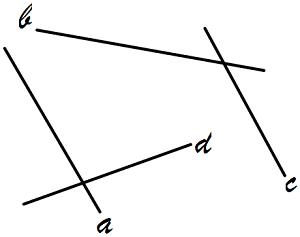### Page 3

#### Question 12 12. Which graph matches the equation below?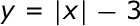### Page 4

#### Question 16 16. What is the slope of the line tangent to the curve given by the equation y = (x-1)²+2 at the point (1,2)?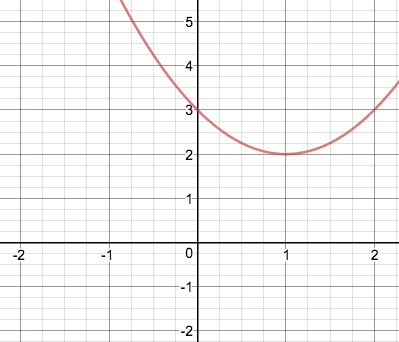#### Question 18 18. Which equation matches the graph below?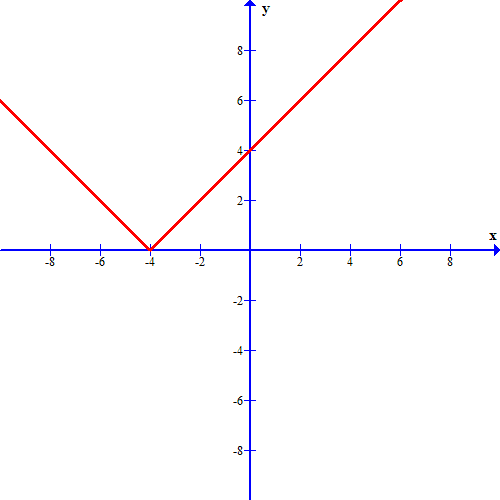#### Question 19 19. Which equation matches the graph below?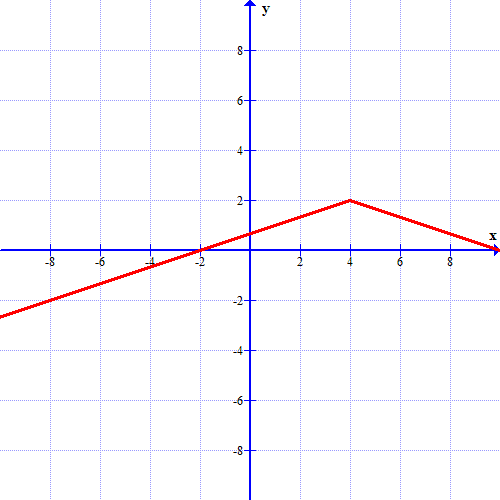### Page 6

#### GRE Quantitative Reasoning - Coordinate Geometry: Tutoring Solution Chapter Exam Instructions

Choose your answers to the questions and click 'Next' to see the next set of questions. You can skip questions if you would like and come back to them later with the yellow "Go To First Skipped Question" button. When you have completed the practice exam, a green submit button will appear. Click it to see your results. Good luck!

Support